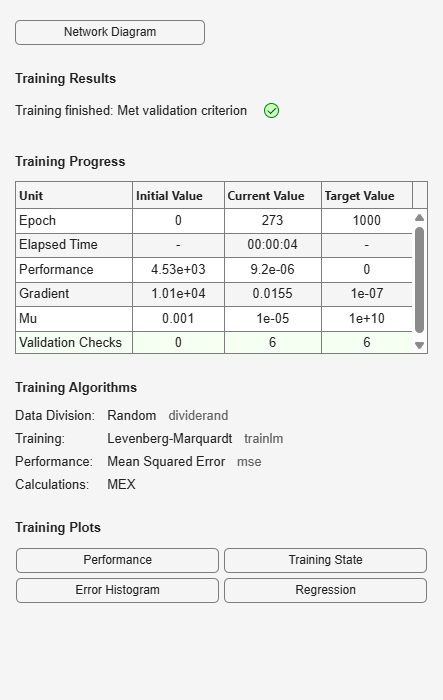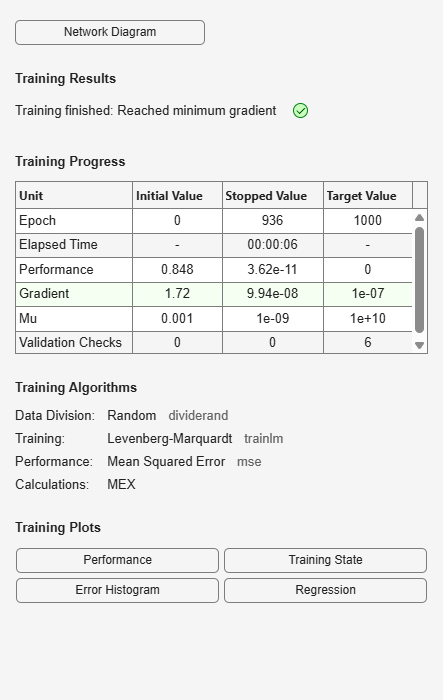## Normalize Errors of Multiple Outputs

The most common performance function used to train neural networks is mean squared error (mse). However, with multiple outputs that have different ranges of values, training with mean squared error tends to optimize accuracy on the output element with the wider range of values relative to the output element with a smaller range.

For instance, here two target elements have very different ranges:

```x = -1:0.01:1; t1 = 100*sin(x); t2 = 0.01*cos(x); t = [t1; t2];```

The range of `t1` is 200 (from a minimum of -100 to a maximum of 100), while the range of `t2` is only 0.02 (from -0.01 to 0.01). The range of `t1` is 10,000 times greater than the range of `t2`.

If you create and train a neural network on this to minimize mean squared error, training favors the relative accuracy of the first output element over the second.

```net = feedforwardnet(5); net1 = train(net,x,t); y = net1(x);```

Here you can see that the network has learned to fit the first output element very well.

```figure(1) plot(x,y(1,:),x,t(1,:))```However, the second element’s function is not fit nearly as well.

```figure(2) plot(x,y(2,:),x,t(2,:))```To fit both output elements equally well in a relative sense, set the `normalization` performance parameter to `'standard'`. This then calculates errors for performance measures as if each output element has a range of 2 (i.e., as if each output element’s values range from -1 to 1, instead of their differing ranges).

```net.performParam.normalization = 'standard'; net2 = train(net,x,t); y = net2(x);```

Now the two output elements both fit well.

```figure(3) plot(x,y(1,:),x,t(1,:))``````figure(4) plot(x,y(2,:),x,t(2,:))```